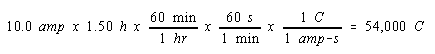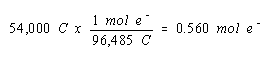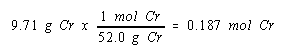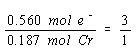Practice Problem 14

Determine the oxidation number of the chromium in an unknown salt if electrolysis of a molten sample of this salt for 1.50 hours with a 10.0-amp current deposits 9.71 grams of chromium metal at the cathode.

Solution

We start, as before, by calculating the number of moles of electrons that passed through the cell during electrolysis.Since we don't know the balanced equation for the reaction at the cathode in this cell, it doesn't seem obvious how we are going to use this information. We therefore write down this result in a conspicuous location, and go back to the original statement of the question, to see what else can be done.

The problem tells us the mass of chromium deposited at the cathode. By now it should be obvious that we need to transform this information into the number of moles of chromium metal generated.We now know the number of moles of chromium metal produced and the number of molus of electrons it took to produce this metal. We might therefore look at the relationship between the moles of electrons consumed in this reaction and the moles of chromium produced.Three moles of electrons are consumed for every mole of chromium metal produced. The only way to explain this is to assume that the net reaction at the cathode involves reduction of Cr3+ ions to chromium metal.

 Cathode (-): Cr3+ + 3 e-Cr

Thus, the oxidation number of chromium in the unknown salt must be +3.